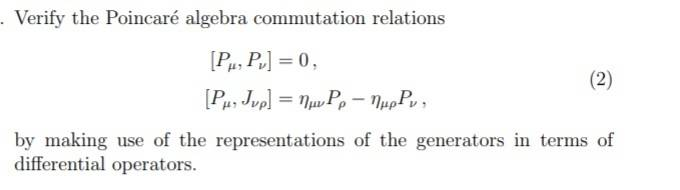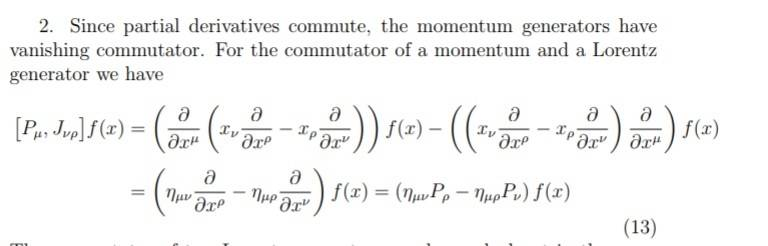# Delta/metric question (context commutator poincare transf.)

binbagsss

## Homework Statement## Homework Equations

[/B]
I believe that ##\frac{\partial x^u}{\partial x^p} =\delta ^u_p ## (1)

##\implies ## (if ##\delta^a_b ## is a tensor, I'm not sure it is?) : ##\frac{\partial x_u}{\partial x^p} = g_{au} \delta ^a_p ## (2)

## The Attempt at a Solution

[/B]
sol attached:because partial derivatives commute four of the terms cancel and I agree with the solution to get the remaining two terms the same, however, I have a delta in place where the solution has a metric so instead I would get(using (2)):

##g_{av}\delta^a_u \frac{\partial}{\partial x^p}-g_{ap}\delta^a_u\frac{\partial}{\partial x^v} ##

#### Attachments

Mentor
2021 Award
The picture already contains the answer. What do you need a metric for in a vector space? It's Euclidean flat, you operate in a tangent space.

binbagsss
The picture already contains the answer. What do you need a metric for in a vector space? It's Euclidean flat, you operate in a tangent space.
sorry, I don't understand your comment.

I believe we have that ##g^u_v=\delta^u_v ## from that ##g_{uv}g^{uv}=1 ##, however this is with one index raised and one lowered whereas the question above has both low, so i dont get how the delta has been replaced with the metric

Mentor
2021 Award
sorry, I don't understand your comment.

I believe we have that ##g^u_v=\delta^u_v ## from that ##g_{uv}g^{uv}=1 ##, however this is with one index raised and one lowered whereas the question above has both low, so i dont get how the delta has been replaced with the metric
What do you care about the metric? You have the differential operators explicitly given, so you can apply them on a suitable function and calculate the commutators. There is no need for ##g\; . \;\frac{\partial x_\mu}{\partial x_\nu}= \delta_{\mu \nu}\,.## It's an ONS.

binbagsss
What do you care about the metric? You have the differential operators explicitly given, so you can apply them on a suitable function and calculate the commutators. There is no need for ##g\; . \;\frac{\partial x_\mu}{\partial x_\nu}= \delta_{\mu \nu}\,.## It's an ONS.
one night stand? what is ONS?

Mentor
2021 Award
Orthonormal system.

binbagsss
i dont care about the metric? the answer has the metric whereas i have deltas

Mentor
2021 Award
Which answer do you mean? I don't know how the ##\eta## are defined and on Wikipedia it seems, that they use ##M_{\mu \nu}=-J_{\mu \nu}## but that's it. I read the ##\eta## as ##\eta_{\mu \nu} = \delta_{\mu \nu}\,.##

A simple calculation will tell. Of course you can assume any basis, but why to complicate things? It's all about the product rule in the end.

binbagsss
Which answer do you mean?[ /QUOTE] 'sol attached'

Mentor
2021 Award
Just differentiate ##f(x)=f(x_1,\ldots,x_4)##.

Homework Helper
Gold Member

## Homework Statement

View attachment 227265

## Homework Equations

[/B]
I believe that ##\frac{\partial x^u}{\partial x^p} =\delta ^u_p ## (1)

##\implies ## (if ##\delta^a_b ## is a tensor, I'm not sure it is?) : ##\frac{\partial x_u}{\partial x^p} = g_{au} \delta ^a_p ## (2)

## The Attempt at a Solution

[/B]
sol attached:View attachment 227264

because partial derivatives commute four of the terms cancel and I agree with the solution to get the remaining two terms the same, however, I have a delta in place where the solution has a metric so instead I would get(using (2)):

##g_{av}\delta^a_u \frac{\partial}{\partial x^p}-g_{ap}\delta^a_u\frac{\partial}{\partial x^v} ##

•